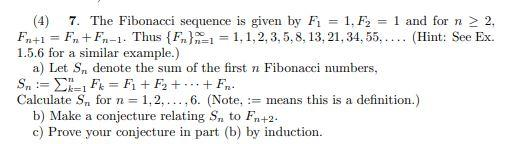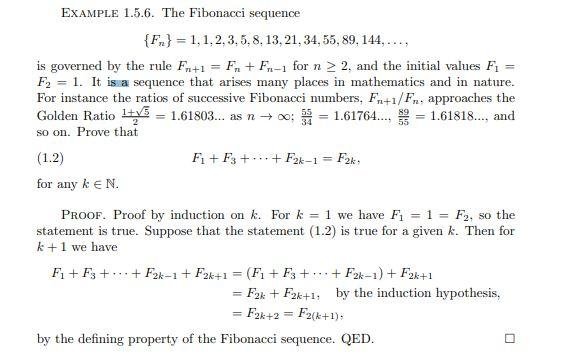# Question PLEASE REFER TO SOLUTION (4) 7. The Fibonacci sequence is given by Fi = 1. F2 = 1 and for n > 2. Fn+1 = F. +Fn-1. Thus {F}=1 = 1,1,2,3,5,8,13, 21, 34, 55, .... (Hint: See Ex. 1.5.6 for a similar example.) a) Let S denote the sum of the first n Fibonacci numbers, Sn:= I= Ft = Fi + F2 + ... + Fr. Calculate S. for n=1,2,..., 6. (Note, := means this is a definition.) b) Make a conjecture relating to Fn+2. c) Prove your conjecture in part (b) by induction. n 2 EXAMPLE 1.5.6. The Fibonacci sequence {Fr} = 1,1,2,3,5,8,13, 21, 34, 55, 89, 144, ..., is governed by the rule Fn+1 = Fn + Fr.-1 for n > 2. and the initial values Fi = F2 = 1. It is a sequence that arises many places in mathematics and in nature. For instance the ratios of successive Fibonacci numbers. Fr+i/Fr. approaches the Golden Ratio + = 1.61803... as n +00: 5 = 1.61764..., 3 = 1.61818..., and so on. Prove that (1.2) Fi + F3 +...+F2k-1 = F2k for any k eN. PROOF. Proof by induction on k. For k = 1 we have Fi = 1 = F2, so the true. Suppose that the statement (1.2) is true for a given k. Then for k+1 we have F1+F3 + ... + F2k-1 + F2k+1 = (F1+F3 + ... + F2k-1) + F2k+1 = F2x + F2k+1: by the induction hypothesis, = F2k+2 = F2(+1), by the defining property of the Fibonacci sequence. QED.